# 网络流之dinic

2019-10-13
5分钟阅读时长

## 网络流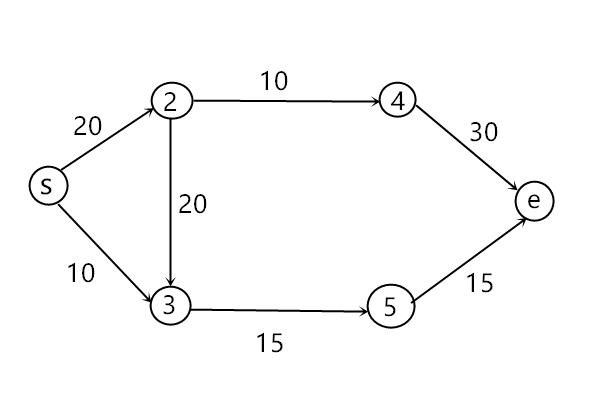## 增广路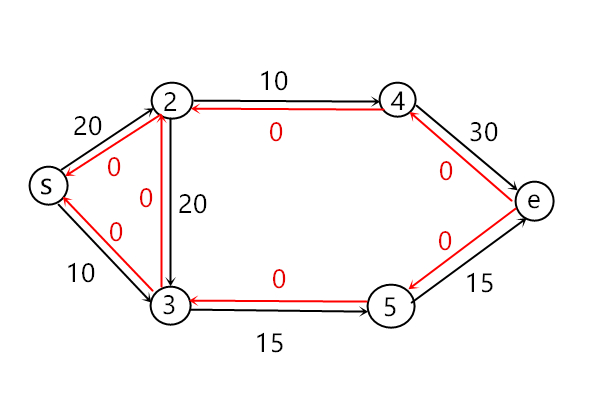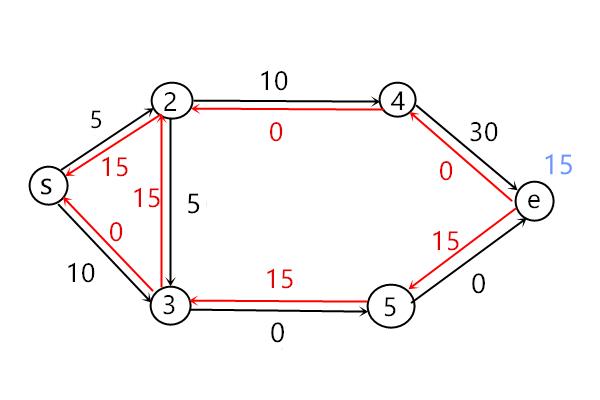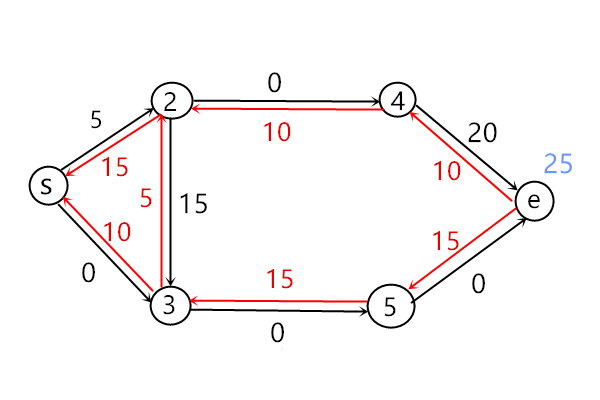## dinic

dinic算法是求解最大流问题的常用算法之一。

dinic将图进行了分层，其实就是用bfs对图进行遍历，为每个顶点标深度。比如上图的s深度为1，顶点2和3深度为2，顶点4和5深度为3，e的深度为4。

dinic规定，在寻找增广路的时候，我们只能走这样的边<u, v>满足顶点v的深度比顶点u的深度大1

#include<bits/stdc++.h>
using namespace std;
typedef long long ll;
typedef pair<int, int> P;
const int maxn = 1e6+5;
const int inf = 0x3f3f3f3f;
const int mod = 1e9+7;

// 用链式前向星来存储图
struct ed
{
int to, val, ne;
}edge[maxn<<1];
// 顶点数n，边数m，源点s，汇点e，加边时的指针tot
int n, m, s, e, tot;

void init()
{
tot = -1;
}

void addEdge(int u, int v, int val)
{
edge[++tot].to = v;
edge[tot].val = val;
}

// 就是最普通的bfs
int bfs()
{
memset(dep, -1, sizeof(dep));
dep[s] = 0;
queue<int> q;
q.push(s);
while(!q.empty())
{
int u = q.front();
q.pop();
{
int v = edge[i].to;
if(dep[v]==-1 && edge[i].val>0) //若这条边还有流量，我们才能走到顶点v，所以才更新v的深度
{
dep[v] = dep[u]+1;
q.push(v);
}
}
}
return (dep[e] != -1); //若dep[e]==-1则表示没有可以到达e的增广路了，算法结束。
}

// 当前顶点u，当前流量flow
// 初始时dfs(s, inf)
int dfs(int u, int flow)
{
// 若当前到达了e，则返回当前flow值
if(u == e)return flow;
{
int v = edge[i].to;
// 若顶点v的深度比u大一，且边<u, v>还有流量
if(dep[v]==dep[u]+1 && edge[i].val)
{
// 递归继续寻找，注意min操作，要传递最小的边权
int a = dfs(v, min(flow, edge[i].val));
if(a>0) //若找到增广路
{
edge[i].val -= a; // 更新正向边和反边的权值
edge[i^1].val += a;
return a;
}
}
}
// 若最后没找到增广路，则返回0
return 0;
}

ll dinic()
{
ll ans = 0;
// 不断地bfs，直到没有增广路
while(bfs())
{
// 找到一条增广路，并且得到这条路能够贡献的流量a。1<<30表示无穷大
int a = dfs(s, (1<<30));
ans += a;
}
return ans;
}

int main()
{
scanf("%d%d%d%d", &n, &m, &s, &e);
init();
for(int i=1;i<=m;i++)
{
int u, v, w;
scanf("%d%d%d", &u, &v, &w);
}
printf("%lld\n", dinic());
return 0;
}


## dinic的优化

dinic算法有三种优化方法。

• 当前弧优化
• 多路增广
• 炸点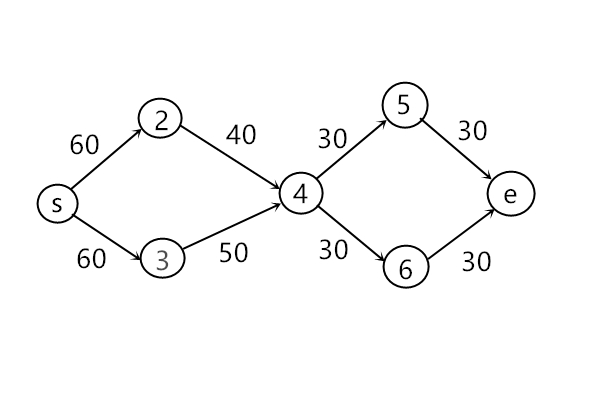#include<bits/stdc++.h>
using namespace std;
typedef long long ll;
typedef pair<int, int> P;
const int maxn = 1e6+5;
const int inf = 0x3f3f3f3f;
const int mod = 1e9+7;

struct ed
{
int to, val, ne;
}edge[maxn<<1];
int n, m, s, e, tot;

void init()
{
tot = -1;
}

void addEdge(int u, int v, int val)
{
edge[++tot].to = v;
edge[tot].val = val;
}

int bfs()
{
memset(dep, -1, sizeof(dep));
dep[s] = 0;
queue<int> q;
q.push(s);
while(!q.empty())
{
int u = q.front();
q.pop();
{
int v = edge[i].to;
if(dep[v]==-1 && edge[i].val>0)
{
dep[v] = dep[u]+1;
q.push(v);
}
}
}
return (dep[e] != -1);
}

int dfs(int u, int flow)
{
if(u == e)return flow;
// rflow用于多路增广，表示流入到顶点u的剩余未流出的流量
int rflow = flow;
// 当前弧优化，通过引用，可以改变cur[i]的值，使得下次遍历到顶点u时，会直接从上次增广的边开始遍历
for(int& i=cur[u]; ~i; i=edge[i].ne)
{
int v = edge[i].to;
if(dep[v]==dep[u]+1 && edge[i].val)
{
int a = dfs(v, min(rflow, edge[i].val));
edge[i].val -= a;
edge[i^1].val += a;
rflow -= a; // 剩余流量要减少
if(rflow<=0)break; // 若没有剩余流量了，就break
}
}
// 若没有一丝流量流出，则表示通过顶点u已经无法增广了，于是炸点，dep可以设置为任何无意义值
if(rflow == flow)
dep[u] = -2;
return flow - rflow; // 返回流出的流量
}

ll dinic()
{
ll ans = 0;
while(bfs())
{
// 新一轮dfs之前要对cur进行初始化
int a = dfs(s, (1<<30));
ans += a;
}
return ans;
}

int main()
{
scanf("%d%d%d%d", &n, &m, &s, &e);
init();
for(int i=1;i<=m;i++)
{
int u, v, w;
scanf("%d%d%d", &u, &v, &w);